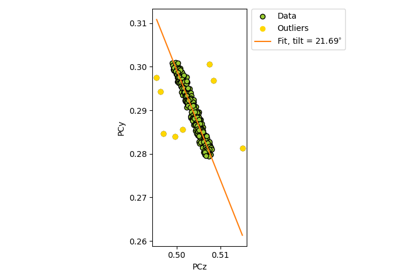# estimate_xtilt#

EBSDDetector.estimate_xtilt(detect_outliers: bool = False, plot: bool = True, degrees: bool = False, return_figure: bool = False, return_outliers: bool = False, figure_kwargs: = None) [source]#

Estimate the tilt about the detector $$X_d$$ axis.

This tilt is assumed to bring the sample plane normal into coincidence with the detector plane normal (but in the opposite direction) .

See the reference frame tutorial for details on the detector sample geometry.

An estimate is found by linear regression of pcz vs. pcy.

Parameters:
detect_outliers

Whether to attempt to detect outliers. If False (default), a linear fit to all points is performed. If True, a robust fit using the RANSAC algorithm is performed instead, which also detects outliers.

plot

Whether to plot data points and the estimated line. Default is True.

degrees

Whether to return the estimated tilt in radians (False, default) or degrees (True).

return_figure

Whether to return the plotted figure. Default is False.

return_outliers

Whether to return a mask with True for PC values considered outliers. Default is False. If True, detect_outliers is assumed to be True and the value passed is not considered.

figure_kwargs

Keyword arguments passed to matplotlib.pyplot.Figure() if plot=True.

Returns:
x_tilt

Estimated tilt about detector $$X_d$$ in radians (degrees=False) or degrees (degrees=True).

outliers

Returned if return_outliers=True, in the shape of navigation_shape.

fig

Returned if plot=True and return_figure=True.

Notes

This method is adapted from Aimo Winkelmann’s function fit_xtilt() in the xcdskd Python package. See for their use of related functions.

## Examples using EBSDDetector.estimate_xtilt#Estimate tilt about the detector x axis

Estimate tilt about the detector x axis